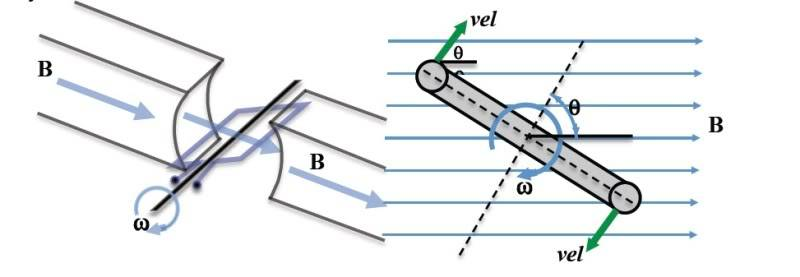# Alternatic Current Derivation

sandy.bridge
Hello all,
I'm trying to find the derivation for the alternating signal. For example,let b=width of loop
a=length of loop
Hence,
$$ε=vLB=vaB=ωraB=\frac{1}{2}ωabB=\frac{1}{2}ωAB$$
I cannot seem to find the derivation of how one gets from this step, to the sinusoidal waveform. Any help is greatly appreciated.